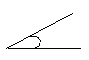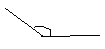Email us to get an instant 20% discount on highly effective K-12 Math & English kwizNET Programs!

#### Online Quiz (WorksheetABCD)

Questions Per Quiz = 2 4 6 8 10

### Grade 3 - Mathematics8.8 Types of Angles

 When two rays meet at a point called vertex an angle is formed.An angle that measures 90 degrees is called right angle.An angle that measures less than 90 degrees is called acute angle.An angle that measures more than 90 degrees is called obtuse angle.Directions: Answer the following questions. Also draw right angle, acute angle, obtuse angle and name the rays, angles and vertex.
 Q 1: The point X in the figure is called the ______SideLineVertex Q 2: An angle that is less than a right angle isacute angleangle lessa right angle Q 3: An angle that is greater than a right angle is calledacute angleobtuse anglea great angle Q 4: What do you call an angle that forms a square corner?right angleobtuse anglesquare angle Question 5: This question is available to subscribers only! Question 6: This question is available to subscribers only!

#### Subscription to kwizNET Learning System offers the following benefits:

• Unrestricted access to grade appropriate lessons, quizzes, & printable worksheets
• Instant scoring of online quizzes
• Progress tracking and award certificates to keep your student motivated
• Unlimited practice with auto-generated 'WIZ MATH' quizzes